# 50A_Current_Sensor_SKU_SEN0098_-DFRobot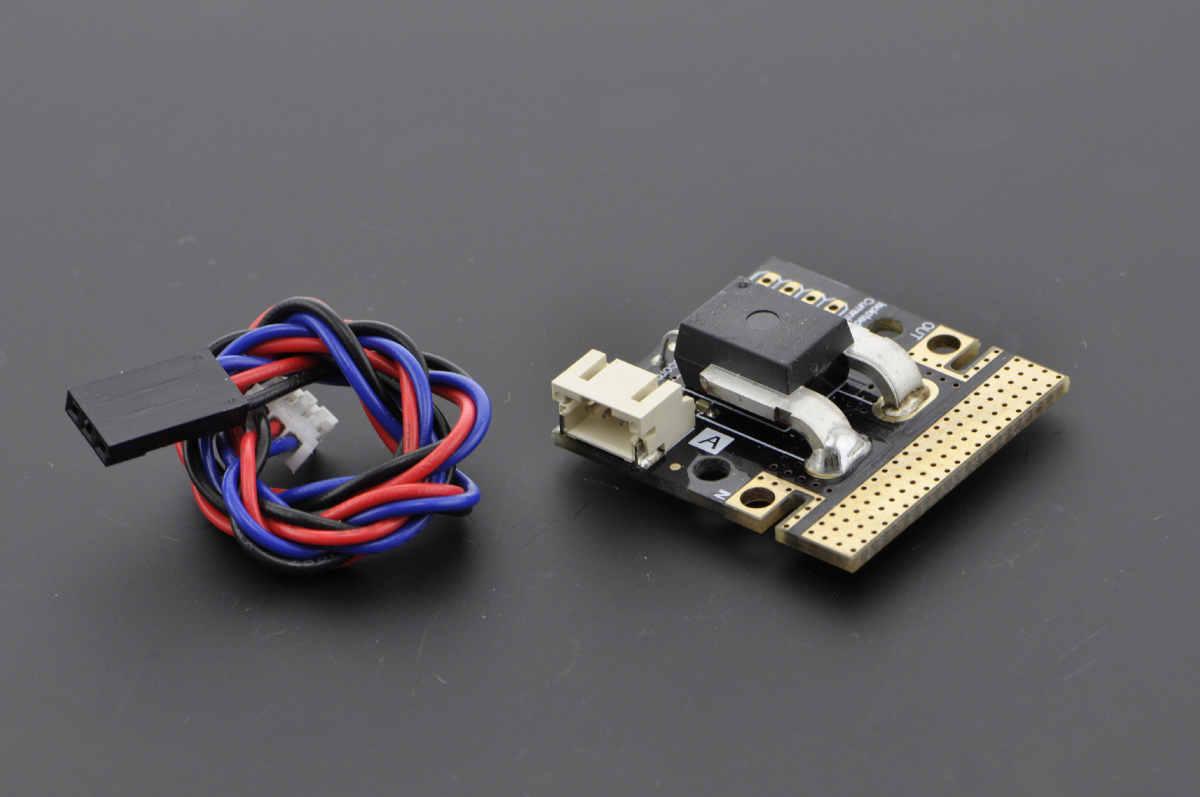## Introduction

This is a breakout board for the fully integrated Hall Effect based linear ACS758 current sensor. The sensor gives precise current measurement for both AC and DC signals.The thickness of the copper conductor allows survival of the device at high over current conditions. The ACS758 outputs an analog voltage output signal that varies linearly with detected current. This current sensor enables you to monitor currents in your project every second for energy saving or circuit protection purposes.

## Specification

• Operating Voltage(analog): 5V
• Peak Measuring Voltage: 3000V(AC), 500V(DC)
• Current Measuring Rang: -50~50A
• Sensitivity: 40 mV/A
• Operating Temperature: -40~150°C
• Dimension: 34x34mm

### PinOut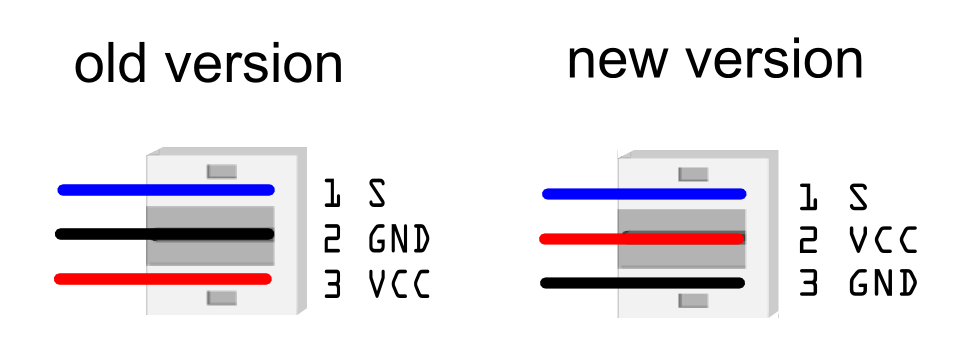## Tutorial

This tutorial is going to test DC and AC current. The result of AC current is its effective value, i.e. the same as an Ammeter reading.

### Connection Diagram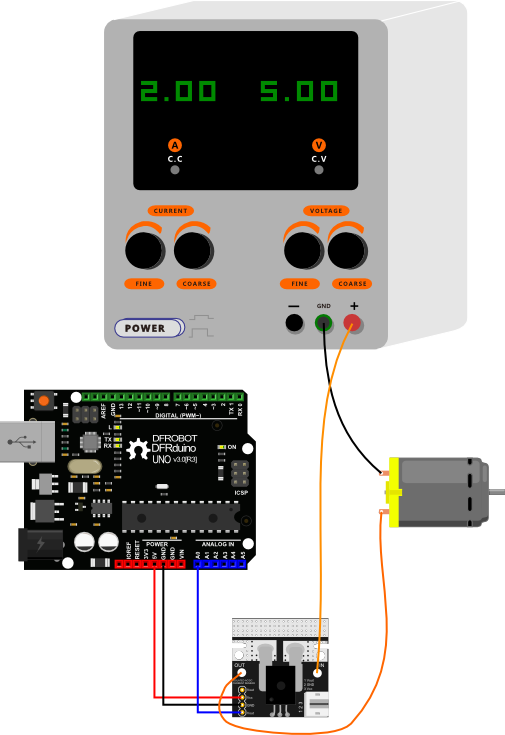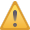DANGER: This diagram can also be applied to AC current connection. But notice that the module should be cascaded on one electric wire ( +/- ). But if you connect it to two electric wires in parallel, you will short the circuit , which is dangerous.

#### Sketch

``````/*
50A Current Sensor(DC)(SKU:SEN0098) Sample Code
This code shows you how to get raw datas from the sensor through Arduino and
convert the raw datas to the value of the current according to the datasheet;

Smoothing algorithm (http://www.arduino.cc/en/Tutorial/Smoothing) is used to
make the outputting current value more reliable;

Created 27 December 2011 @Barry Machine @www.dfrobot.com
*/

int index = 0;                  // the index of the current reading
float total = 0;                  // the running total
float average = 0;                // the average

float currentValue = 0;

void setup()
{
Serial.begin(115200);
}
void loop()
{
//Data processing:510-raw data from analogRead when the input is 0;
// 5-5v; the first 0.04-0.04V/A(sensitivity); the second 0.04-offset val;

index = index   1;
index = 0;
average = total/numReadings;   //Smoothing algorithm (http://www.arduino.cc/en/Tutorial/Smoothing)
currentValue= average;
Serial.println(currentValue);
delay(10);
}``````

#### Result

After uploading the sample sketch, open the serial monitor to monitor your results. You may find that when the current is 0A, you may found the reading is not 0A. In this case, you need to revise the code for some small calibrations.These are the steps:

1 Check the initial current value when the input is 0A: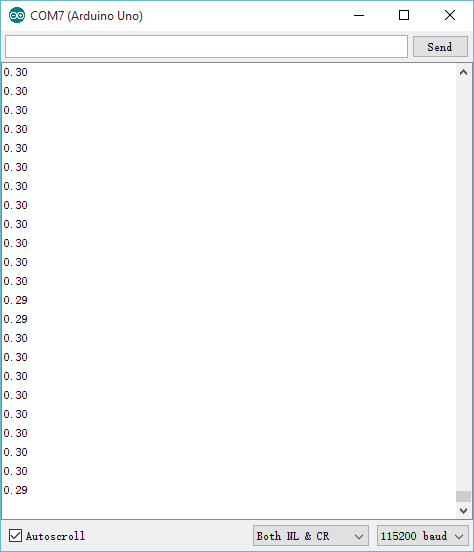2 Caculate the initial current value:

`-0.34 = -0.04 - ( 0.30 );`

3 Revise the sample sketch:

``    readings[index] = (readings[index]-510)*5/1024/0.04-0.34;//``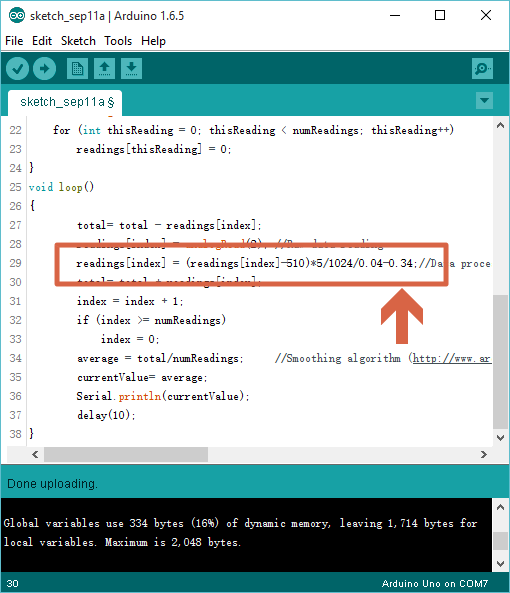4 Upload your new sketch again, then you will found the readinga are around 0A, if not, please do the steps above several times to make it.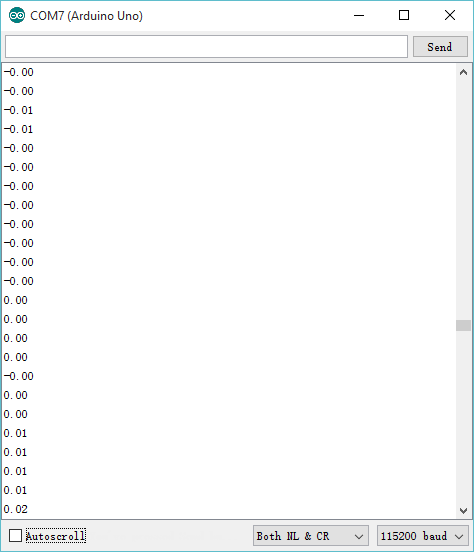5 As shown above, it can be applied to detect the current value accurately, and output analog voltage signals.

#### Sketch

``````float reading = 0;
float currentValue = 0;

void setup(){
Serial.begin(115200);
}
void loop() {
currentValue = (reading - 510) * 5 / 1024 / 0.04 - 0.34;
Serial.println(currentValue);
delay(2);
}``````

### Result of AC current

I tested with a lamp @220V~, and got the result shown below: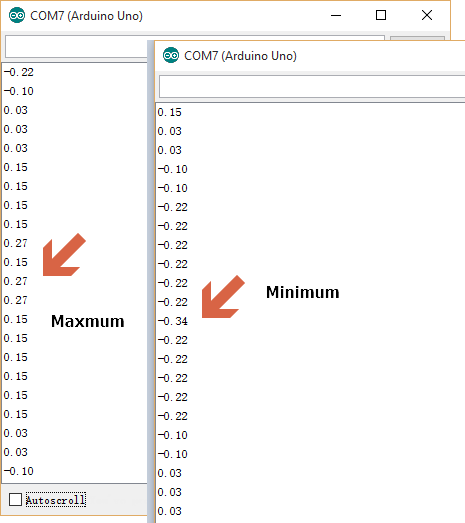Since AC current here is a Sine wave, you want to focus on the amplitude of your waveform. You can read the extreme data which are the maxmum value 0.27 and the minimum value -0.34, and use the maxmum absolute value one as the ( Effective) AC current. In this case its .34

## FAQ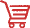Get Gravity: Analog 50A Current Sensor from DFRobot Store or DFRobot Distributor.